# gtcc

Extract gammatone cepstral coefficients, log-energy, delta, and delta-delta

## Syntax

``coeffs = gtcc(audioIn,fs)``
``coeffs = gtcc(___,Name=Value)``
``[coeffs,delta,deltaDelta,loc] = gtcc(___)``
``gtcc(___)``

## Description

example

````coeffs = gtcc(audioIn,fs)` returns the gammatone cepstral coefficients (GTCCs) for the audio input, sampled at a frequency of `fs` Hz.```

example

````coeffs = gtcc(___,Name=Value)` specifies options using one or more name-value arguments.```

example

````[coeffs,delta,deltaDelta,loc] = gtcc(___)` also returns the delta, delta-delta, and location in samples corresponding to each window of data. You can specify an input combination from any of the previous syntaxes.```

example

````gtcc(___)` with no output arguments plots the gammatone cepstral coefficients. Before plotting, the coefficients are normalized to have mean 0 and standard deviation 1. If the input is in the time domain, the coefficients are plotted against time.If the input is in the frequency domain, the coefficients are plotted against frame number.If the log-energy is extracted, then it is also plotted. ```

## Examples

collapse all

Get the gammatone cepstral coefficients for an audio file using default settings.

```[audioIn,fs] = audioread("Counting-16-44p1-mono-15secs.wav"); [coeffs,~,~,loc] = gtcc(audioIn,fs);```

Plot the normalized coefficients.

`gtcc(audioIn,fs)`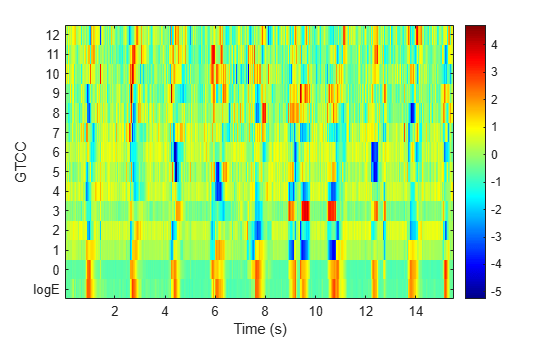Read in an audio file.

`[audioIn,fs] = audioread("Turbine-16-44p1-mono-22secs.wav");`

Calculate 20 GTCCs using filters equally spaced on the ERB scale between `hz2erb(62.5)` and `hz2erb(12000)`. Calculate the coefficients using 50 ms periodic Hann windows with 25 ms overlap. Replace the 0th coefficient with the log-energy. Use time-domain filtering.

```[coeffs,~,~,loc] = gtcc(audioIn,fs, ... NumCoeffs=20, ... FrequencyRange=[62.5,12000], ... Window=hann(round(0.05*fs),"periodic"), ... OverlapLength=round(0.025*fs), ... LogEnergy="replace", ... FilterDomain="time");```

Plot the normalized coefficients.

```gtcc(audioIn,fs, ... NumCoeffs=20, ... FrequencyRange=[62.5,12000], ... Window=hann(round(0.05*fs),"periodic"), ... OverlapLength=round(0.025*fs), ... LogEnergy="replace", ... FilterDomain="time")```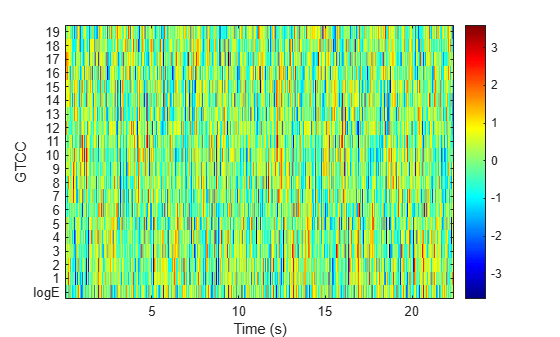Read in an audio file and convert it to a frequency representation.

```[audioIn,fs] = audioread("Rainbow-16-8-mono-114secs.wav"); win = hann(1024,"periodic"); S = stft(audioIn,"Window",win,"OverlapLength",512,"Centered",false);```

To extract the gammatone cepstral coefficients, call `gtcc` with the frequency-domain audio. Ignore the log-energy.

`coeffs = gtcc(S,fs,"LogEnergy","Ignore");`

In many applications, GTCC observations are converted to summary statistics for use in classification tasks. Plot a probability density function for one of the gammatone cepstral coefficients to observe its distributions.

```nbins = 60; coefficientToAnalyze =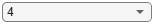4; histogram(coeffs(:,coefficientToAnalyze+1),nbins,'Normalization','pdf') title(sprintf("Coefficient %d",coefficientToAnalyze))```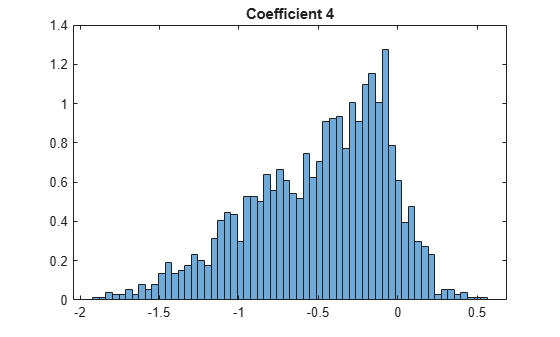## Input Arguments

collapse all

Input signal, specified as a vector, matrix, or 3-D array.

If `FilterDomain` is set to `"frequency"` (default), then `audioIn` can be real or complex.

• If `audioIn` is real, it is interpreted as a time-domain signal and must be a column vector or a matrix. Columns of the matrix are treated as independent audio channels.

• If `audioIn` is complex, it is interpreted as a frequency-domain signal. In this case, `audioIn` must be an L-by-M-by-N array, where L is the number of DFT points, M is the number of individual spectra, and N is the number of individual channels.

If `FilterDomain` is set to `"time"`, then `audioIn` must be a real column vector or matrix. Columns of the matrix are treated as independent audio channels.

Data Types: `single` | `double`
Complex Number Support: Yes

Sample rate of the input signal in Hz, specified as a positive scalar.

Data Types: `single` | `double`

### Name-Value Arguments

Specify optional pairs of arguments as `Name1=Value1,...,NameN=ValueN`, where `Name` is the argument name and `Value` is the corresponding value. Name-value arguments must appear after other arguments, but the order of the pairs does not matter.

Before R2021a, use commas to separate each name and value, and enclose `Name` in quotes.

Example: `coeffs = gtcc(audioIn,fs,LogEnergy="replace")` returns gammatone cepstral coefficients for the audio input signal sampled at `fs` Hz. For each analysis window, the first coefficient in the `coeffs` vector is replaced with the log energy of the input signal.

Window applied in time domain, specified as a real vector. The number of elements in the vector must be in the range `[1,size(audioIn,1)]`. The number of elements in the vector must also be greater than `OverlapLength`.

Data Types: `single` | `double`

Number of samples overlapped between adjacent windows, specified as an integer in the range [0, `numel(Window)`). If unspecified, `OverlapLength` defaults to `round(0.02*fs)`.

Data Types: `single` | `double`

Number of coefficients returned for each window of data, specified as an integer in the range [2, v]. v is the number of valid passbands. If unspecified, `NumCoeffs` defaults to `13`.

The number of valid passbands is defined as the number of ERB steps (ERBN) in the frequency range of the filter bank. The frequency range of the filter bank is specified by `FrequencyRange`.

Data Types: `single` | `double`

Domain in which to apply filtering, specified as `"frequency"` or `"time"`. If unspecified, `FilterDomain` defaults to `"frequency"`.

Data Types: `string` | `char`

Frequency range of gammatone filter bank in Hz, specified as a two-element row vector of increasing values in the range [0, `fs`/2]. If unspecified, `FrequencyRange` defaults to ```[50, fs/2]```

Data Types: `single` | `double`

Number of bins used to calculate the discrete Fourier transform (DFT) of windowed input samples. The FFT length must be greater than or equal to the number of elements in the `Window`.

Data Types: `single` | `double`

Type of nonlinear rectification applied prior to the discrete cosine transform, specified as `'log'` or `'cubic-root'`.

Data Types: `char` | `string`

Number of coefficients used to calculate the delta and the delta-delta values, specified as an odd integer greater than two. If unspecified, `DeltaWindowLength` defaults to `9`.

Deltas are computed using the `audioDelta` function.

Data Types: `single` | `double`

Log energy usage, specified as `"append"`, `"replace"`, or `"ignore"`. If unspecified, `LogEnergy` defaults to `"append"`.

• `"append"` –– The function prepends the log energy to the coefficients vector. The length of the coefficients vector is 1 + `NumCoeffs`.

• `"replace"` –– The function replaces the first coefficient with the log energy of the signal. The length of the coefficients vector is `NumCoeffs`.

• `"ignore"` –– The function does not calculate or return the log energy.

Data Types: `char` | `string`

## Output Arguments

collapse all

Gammatone cepstral coefficients, returned as an L-by-M matrix or an L-by-M-by-N array, where:

• L –– Number of analysis windows the audio signal is partitioned into. The input size, `Window`, and `OverlapLength` control this dimension: ```L = floor((size(audioIn,1) − numel(Window)))/(numel(Window) − OverlapLength) + 1```.

• M –– Number of coefficients returned per frame. This value is determined by `NumCoeffs` and `LogEnergy`.

When `LogEnergy` is set to:

• `"append"` –– The function prepends the log energy value to the coefficients vector. The length of the coefficients vector is 1 + `NumCoeffs`.

• `"replace"` –– The function replaces the first coefficient with the log energy of the signal. The length of the coefficients vector is `NumCoeffs`.

• `"ignore"` –– The function does not calculate or return the log energy. The length of the coefficients vector is `NumCoeffs`.

• N –– Number of input channels (columns). This value is `size(audioIn,2)`.

Data Types: `single` | `double`

Change in coefficients from one analysis window to another, returned as an L-by-M matrix or an L-by-M-by-N array. The `delta` array is the same size and data type as the `coeffs` array. See `coeffs` for the definitions of L, M, and N.

Data Types: `single` | `double`

Change in `delta` values, returned as an L-by-M matrix or an L-by-M-by-N array. The `deltaDelta` array is the same size and data type as the `coeffs` and `delta` arrays. See `coeffs` for the definitions of L, M, and N.

Data Types: `single` | `double`

Location of last sample in each analysis window, returned as a column vector with the same number of rows as `coeffs`.

Data Types: `single` | `double`

## Algorithms

collapse all

The `gtcc` function splits the entire data into overlapping segments. The length of each analysis window is determined by `Window`. The length of overlap between analysis windows is determined by `OverlapLength`. The algorithm to determine the gammatone cepstral coefficients depends on the filter domain, specified by `FilterDomain`. The default filter domain is frequency.

### Frequency-Domain Filtering

Gammatone cepstrum coefficients are popular features extracted from speech signals for use in recognition tasks. In the source-filter model of speech, cepstral coefficients are understood to represent the filter (vocal tract). The vocal tract frequency response is relatively smooth, whereas the source of voiced speech can be modeled as an impulse train. As a result, the vocal tract can be estimated by the spectral envelope of a speech segment.

The motivating idea of gammatone cepstral coefficients is to compress information about the vocal tract (smoothed spectrum) into a small number of coefficients based on an understanding of the cochlea. Although there is no hard standard for calculating the coefficients, the basic steps are outlined by the diagram.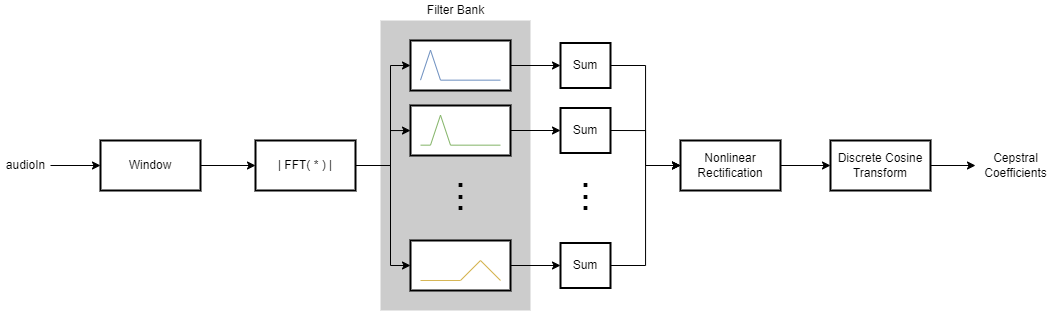The default gammatone filter bank is composed of gammatone filters spaced linearly on the ERB scale between 50 and 8000 Hz. The filter bank is designed by `designAuditoryFilterBank`.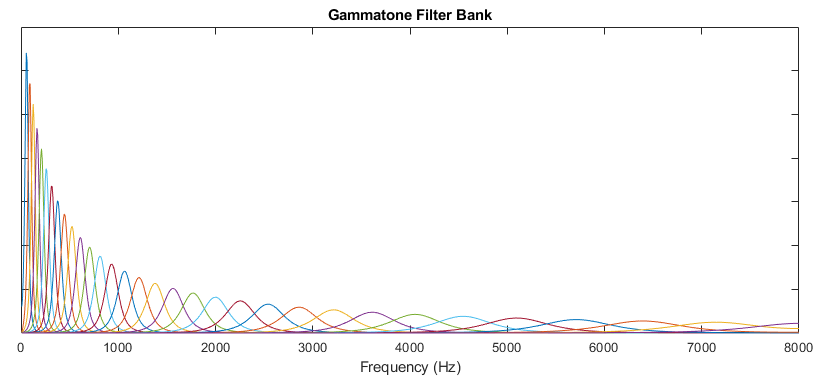The information contained in the zeroth gammatone cepstral coefficient is often augmented with or replaced by the log energy. The log energy calculation depends on the input domain.

If the input is a time-domain signal, the log energy is computed using the following equation:

`$\mathrm{log}E=\mathrm{log}\left(\text{sum}\left({x}^{2}\right)\right)$`

If the input is a frequency-domain signal, the log energy is computed using the following equation:

`$\mathrm{log}E=\mathrm{log}\left(\text{sum}\left({|x|}^{2}\right)/FFTLength\right)$`

### Time-Domain Filtering

If `FilterDomain` is specified as `"time"`, the `gtcc` function uses the `gammatoneFilterBank` to apply time-domain filtering. The basic steps of the `gtcc` algorithm are outlined by the diagram.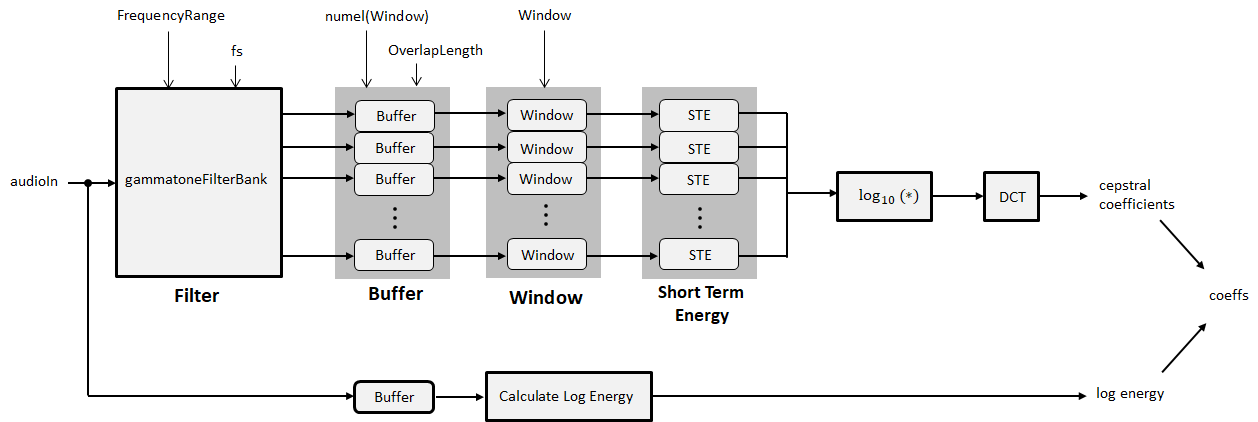The `FrequencyRange` and sample rate (`fs`) parameters are set on the filter bank using the name-value pairs input to the `gtcc` function. The number of filters in the gammatone filter bank is defined as ```hz2erb(FrequencyRange(2)) − hz2erb(FrequencyRange(1))```.This roughly corresponds to placing a gammatone filter every 0.9 mm in the cochlea.

The output from the gammatone filter bank is a multichannel signal. Each channel output from the gammatone filter bank is buffered into overlapped analysis windows, as specified by the `Window` and `OverlapLength` parameters. The energy for each analysis window of data is calculated. The STE of the channels are concatenated. The concatenated signal is then passed through a logarithm function and transformed to the cepstral domain using a discrete cosine transform (DCT).

The log-energy is calculated on the original audio signal using the same buffering scheme applied to the gammatone filter bank output.

 Shao, Yang, Zhaozhang Jin, Deliang Wang, and Soundararajan Srinivasan. "An Auditory-Based Feature for Robust Speech Recognition." IEEE International Conference on Acoustics, Speech and Signal Processing. 2009.

 Valero, X., and F. Alias. "Gammatone Cepstral Coefficients: Biologically Inspired Features for Non-Speech Audio Classification." IEEE Transactions on Multimedia. Vol. 14, Issue 6, 2012, pp. 1684–1689.